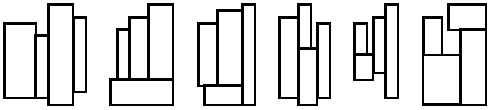시간 제한 메모리 제한 제출 정답 맞은 사람 정답 비율
1 초 512 MB 0 0 0 0.000%

## 문제Figure 1: The six basic layouts of four rectangles

Four rectangles are given. Find the smallest enclosing (new) rectangle into which these four may be fitted without overlapping. By smallest rectangle we mean the one with the smallest area.

All four rectangles should have their sides parallel to the corresponding sides of the enclosing rectangle. Figure 1 shows six ways to fit four rectangles together. These six are the only possible basic layouts, since any other layout can be obtained from a basic layout by rotation or reflection.

There may exist several different enclosing rectangles fulfilling the requirements, all with the same area. You have to produce all such enclosing rectangles.

## 입력

The input consists of four lines. Each line describes one given rectangle by two positive integers: the lengths of the sides of the rectangle. Each side of a rectangle is at least 1 and at most 50.

## 출력

The output should contain one line more than the number of solutions. The first line contains a single integer: the minimum area of the enclosing rectangles (Subtask A). Each of the following lines contains one solution described by two numbers p and q with p<=q (Subtask B). These lines must be sorted in ascending order of p, and must all be different.

## 예제 입력 1

1 2
2 3
3 4
4 5


## 예제 출력 1

40
4 10
5 8